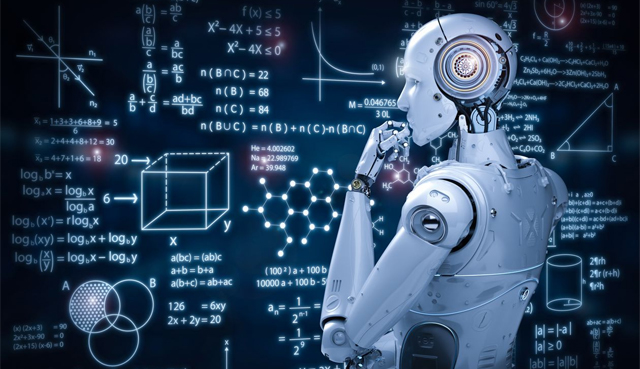# It All Started With AI …and Here We AreWhatsApp shared by Ghulam Mustafa, Ph.D, USA: It started with AI: I started working on ML about 10 years ago as a natural extension of my interest in probability and statistical inference. I learned of the Occam’s razor, that simpler models are preferred over complex which led to bias-variance trade-off and to Kullback-Liebler variational inference.

In deep AI, I learned that the cost is related to energy. we want to minimize cost (and hence energy) via back propagation (and gradient descent), which led to Lagrange optimization -which is the direct outcome of Euler-Lagrange formulation in mechanics.

Most energy based models in AI rely on these concepts and make extensive use of Partition Function to compute the Gibbs measure. All these are founded in Statistical Mechanics. Of particular interest are Spin Glasses like the Ising model or closely related Sherrington-Kirkpatrick, of how local changes give rise to large scale behavior, which led to the mean fields for capturing aggregate behaviors. This is done in the Hamiltonian setting, as Newtonian formulism runs out of gas at this point (and that I have a bone to pick with Isaac).

All related to the thermodynamic equilibrium (equal a priori distribution) and the ideal gas models of Maxwell and Boltzmann, with Gibbs providing statistical frame work, which I learned is a slippery slope, as it didn’t satisfactorily explain the Poincare Recurrence (that, given enough time, system will return to its initial configuration, albeit with zero measure).